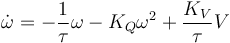# Booz

## Simulator

### Flight dynamic model

in files : sw/simulator/booz_flight_model.c

Hover point hypothesis is assumed, ie each rotor only produces a force normal to the rotor and a moment along the same axis, both proportional to the square of the prop rotational speed.

#### Rotors dynamic

Motor dynamics is simulated. modelisation by a Riccati equation :$\dot{\omega} = - \frac{1}{\tau}\omega - K_{Q}\omega^2 + \frac{K_{V}}{\tau}V$

### Sensors model

in files : sw/simulator/booz_sensors_model.c

### Display

can use flightgear as a 3D display

example command line :

fgfs --fdm=null --native-gui=socket,in,30,,5501,udp --aircraft=bo105

Associated command line options for the simulator : --fg_host and --fg_port

### Interactive control

The radio control can be replaced by a joystick.

the joystick device can be specified with the --js_dev option